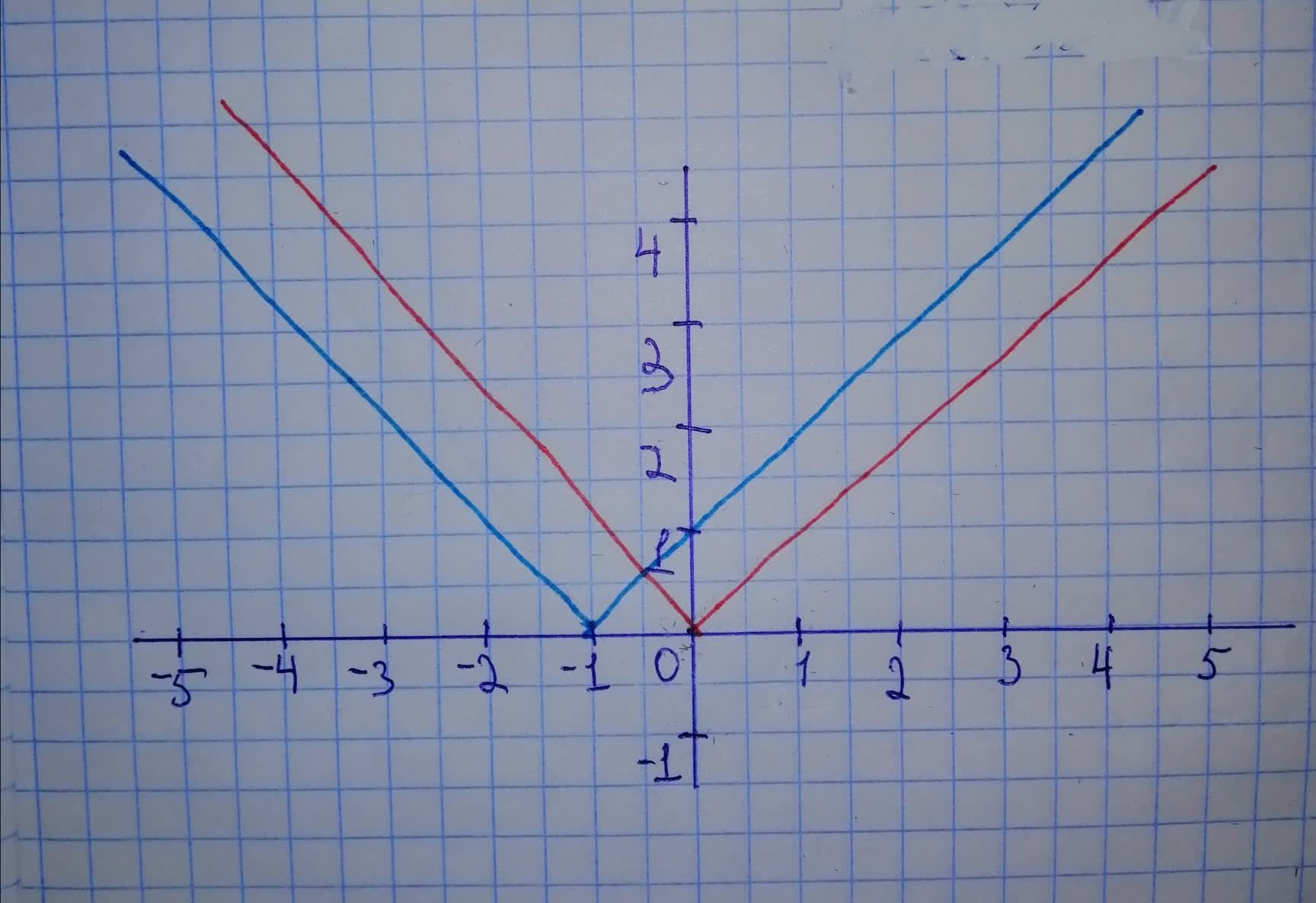# Sketch a graph of the function. Use transformations of functions whenever possible. f(x)=|x + 1|remolatg 2020-12-22 Answered
Sketch a graph of the function. Use transformations of functions whenever possible.
You can still ask an expert for help

• Questions are typically answered in as fast as 30 minutes

Solve your problem for the price of one coffee

• Math expert for every subject
• Pay only if we can solve itNichole Watt
First graph function $y=|x|$ Then, to obtain graph of function , do following transformations: - shift 1 unit to the left On graph: Red - $y=|x|$ Blue -